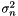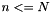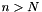#Boost C++ Libraries

...one of the most highly regarded and expertly designed C++ library projects in the world.

This is the documentation for an old version of Boost. Click here to view this page for the latest version.

## Struct template lazy_rolling_variance_impl

boost::accumulators::impl::lazy_rolling_variance_impl — Immediate (lazy) calculation of the rolling variance.

## Synopsis

```// In header: <boost/accumulators/statistics/rolling_variance.hpp>

template<typename Sample>
struct lazy_rolling_variance_impl {
// types
typedef numeric::functional::fdiv< Sample, std::size_t, void, void >::result_type result_type;

// construct/copy/destruct
lazy_rolling_variance_impl(dont_care);

// public member functions
template<typename Args> result_type result(Args const &) const;
};```

## Description

Calculation of sample varianceis done as follows, see also http://en.wikipedia.org/wiki/Algorithms_for_calculating_variance. For a rolling window of size, when, the variance is computed according to the formula

Equation 1.11.When, the sample variance over the window becomes:

Equation 1.12.### `lazy_rolling_variance_impl` public construct/copy/destruct

1. `lazy_rolling_variance_impl(dont_care);`

### `lazy_rolling_variance_impl` public member functions

1. `template<typename Args> result_type result(Args const & args) const;`
 Copyright © 2005, 2006 Eric Niebler Distributed under the Boost Software License, Version 1.0. (See accompanying file LICENSE_1_0.txt or copy at http://www.boost.org/LICENSE_1_0.txt)